# Tolerancing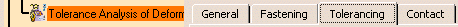This page deals with the options concerning:

## Conversion Range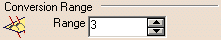Defines the conversion range options:

### Range

Defines the range value to switch between tolerance interval and statistics law.
For example, with a range value of 3 and applied to a normal law, 99.73% of measures contained in the tolerance interval represent the statistics law field.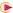By default, the range is 3.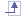## Tolerance Translation Reduction Coefficient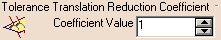Defines the tolerance translation reduction coefficient options:

### Coefficient Value

Defines the coefficient value to translate functional tolerances due to form uncertainty in deviation point grid.By default, the coefficient value is 1.## Minimum VarianceDefines the minimum variance options:

### Minimum Variance

Defines the percentage value of minimum variance to translate functional tolerances.By default, the minimum variance is 50%.